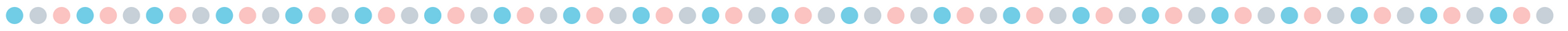# Valentine's Day Math Mats (Free printable)

Ways to Use the Math Mats:
• With a dry erase marker only: (The fine tip dry erase markers work better)
• You announce two numbers and the students write the numbers in each turkey, then the students complete the addition or subtraction sentence. or
• You announce two numbers and the students make dots in each turkey to represent the numbers, then the students complete the addition or subtraction sentence.
• With a dry erase marker and erasers:
• You can announce two numbers and the students put the number of erasers in each turkey, then the students complete the addition or subtraction sentence
• With a dry erase marker and dice:
• Students roll their dice, then place each die in the turkey, then the students complete the addition or subtraction sentence.
• You could use dice with 4, 6, 8,  10, 12, or 20 sided dice to differentiate your instruction.
Supplies: